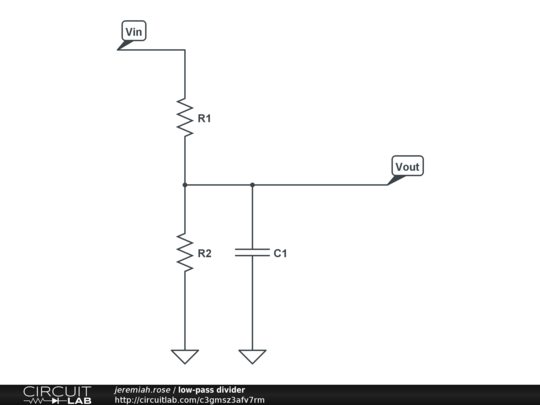# Electronic – Divider and lowpass combined

I am using the following circuit for two purposes:

• As a voltage divider, to reduce DC sensor output to a range suitable for my ADC.
• As a lowpass filter, to remove high frequency noise from the signal.It works great, but my choice of component values has largely been a matter of trial and error, so I have a few questions:

1. What is this type of circuit called? Is it a low-pass filter, a voltage divider or both?
2. What is the equation for \$f_c\$ (cutoff frequency) for this circuit and how is it derived?
3. What is the output impedance \$Z_{out}\$ of the circuit in terms of \$R_1\$, \$R_2\$ and \$C_1\$?
4. If the design requires a specific \$f_c\$, \$Z_{out}\$ and DC \$\frac{V_{out}}{V_{in}}\$, is it possible to derive simple equations for \$R_1\$, \$R_2\$ and \$C_1\$ in terms of these?

2. $$\fc=\frac{(R_1+R_2)\sqrt{(10^{3/10}-1)}}{2\pi*R_1*R_2*C_1}\approx \frac{(R_1+R_2)}{2\pi*R_1*R_2*C_1} \$$
3. $$\R_1//R_2//C_1 \$$